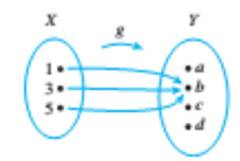Chapter 7.1, Problem 2ES### Discrete Mathematics With Applicat...

5th Edition
EPP + 1 other
ISBN: 9781337694193

#### Solutions

Chapter
Section### Discrete Mathematics With Applicat...

5th Edition
EPP + 1 other
ISBN: 9781337694193
Textbook Problem
1 views

# Let X = { 1 ,   3 ,   5 } and Y = { a ,   b ,   c ,   d } . Define g :   X → Y by the following arrow diagram.What the domain of g and the co-domain of g. Find g ( 1 ) ,   g ( 3 ) , and g ( 5 ) ? What is the range of g? Is 3 an inverse image of a? Is 1 an inverse image of b? What is the inverse image of b? of c? Represent g as a set of ordered pairs.

To determine

(a)

Write the domain of g and the co-domain of g.

Explanation

Given information:

Concept used:

f(x)=yHere, x:domainy:codomain

Calculation:

Consider the following sets as,

X={1,3,5} and Y={a,b,c,d}.

Also, a function g:XY defined as follows.

The definition for domain, Codomain and range of a function are as follows:

Domain: The domain of a function g(x) is the set of all values for which the function is defined

To determine

(b)

Find g(1),g(3) and g(5).

To determine

(c)

What is the range of g

To determine

(d)

Is 3 an inverse image of a ? Is 1 an inverse image of b ?

To determine

(e)

What is the inverse image of b ? Of c ?

To determine

(f)

Represent g as a set of ordered pairs.

### Still sussing out bartleby?

Check out a sample textbook solution.

See a sample solution

#### The Solution to Your Study Problems

Bartleby provides explanations to thousands of textbook problems written by our experts, many with advanced degrees!

Get Started